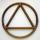# Ratio + triangle - examples

On solving problems and tasks with proportionally we recommend hint rule of three. Rule of three (proportionality) help solve examples of direct and inverse proportionality. Three members makes possible to calculate the fourth - unknown member.

1. Lateral surface areaThe ratio of the area of the base of the rotary cone to its lateral surface area is 3: 5. Calculate the surface and volume of the cone, if its height v = 4 cm.
2. GardenArea of a square garden is 6/4 of triangle garden with sides 56 m, 35 m, and 35 m. How many meters of fencing need to fence a square garden?
3. Gimli GliderAircraft Boeing 767 lose both engines at 42000 feet. The plane captain maintain optimum gliding conditions. Every minute, lose 1910 feet and maintain constant speed 211 knots. Calculate how long takes to plane from engines failure to hit ground. Calculate
4. ObserverThe observer sees straight fence 100 m long in 30° view angle. From one end of the fence is 153 m. How far is it from the another end of the fence?
5. Right triangleLegs of right are in ratio a:b = 6:8. Hypotenuse has a length of 61 cm. Calculate the perimeter and area of the triangle.
6. ISO triangleCalculate the area of an isosceles triangle KLM if the length of its sides are in the ratio k:l:m = 4:4:3 and has perimeter 377 mm.
7. StairwayWhat angle rising stairway if step height in 17 cm and width 27 cm?
8. RhombusInternal angles of rhombus is in ratio 2:3. How many times is the shorter diagonal longer than side of rhombus?
9. MO - trianglesOn the AB and AC sides of the triangle ABC lies successive points E and F, on segment EF lie point D. The EF and BC lines are parallel and is true this ratio FD:DE = AE:EB = 2:1. The area of ABC triangle is 27 hectares and line segments EF, AD, and DB se
10. Trapezoid - diagonalTrapezoid has a length of diagonal AC corssed with diagonal BD in the ratio 2:1. The triangle created by points A, cross point of diagonals S and point D has area 164 cm2. What is the area of the trapezoid?
11. Triangle in circleVertices of the triangle ABC lies on a circle with radius 3 so that it is divided into three parts in the ratio 4:4:4. Calculate the circumference of the triangle ABC.
12. Triangle anglesThe angles α, β, γ in triangle ABC are in the ratio 6:2:6. Calculate size of angles.Average climb of the road is given by ratio 1:15. By what angle road average climb?
14. Circumferential angleVertices of the triangle ΔABC lies on circle and divided it into arcs in the ratio 2:2:9. Determine the size of the angles of the triangle ΔABC.
15. Sun raysIf the sun's rays are at an angle 60° then famous Great Pyramid of Egypt (which is now high 137.3 meters) has 79.3 m long shadow. Calculate current height of neighboring chefren pyramid whose shadow is measured at the same time 78.8 m and the current hei
16. Trapezoid RTThe plot has a shape of a rectangular trapezium ABCD, where ABIICD with a right angle at the vertex B. side AB has a length 36 m. The lengths of the sides AB and BC are in the ratio 12:7. Lengths of the sides AB and CD are a ratio 3:2. Calculate consumptio
17. Angles ratioIn a triangle ABC true relationship c is less than b and b is less than a. Internal angles of the triangle are in the ratio 5:4:9. The size of the internal angle beta is:
18. Isosceles triangleWhat are the angles of an isosceles triangle ABC if its base is long a=5 m and has an arm b=4 m.
19. RT and ratioA right triangle whose legs are in a ratio 6:12 has hypotenuse 68 m long. How long are its legs?
20. Isosceles triangleThe perimeter of an isosceles triangle is 112 cm. The length of the arm to the length of the base is at ratio 5:6. Find the triangle area.

Do you have an interesting mathematical example that you can't solve it? Enter it, and we can try to solve it.

To this e-mail address, we will reply solution; solved examples are also published here. Please enter e-mail correctly and check whether you don't have a full mailbox.